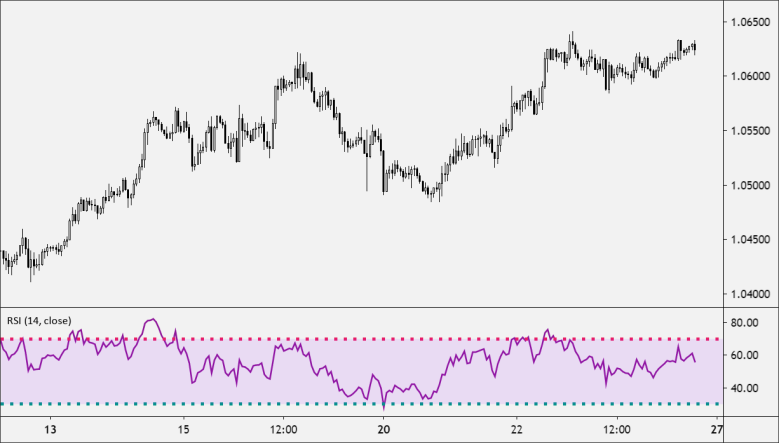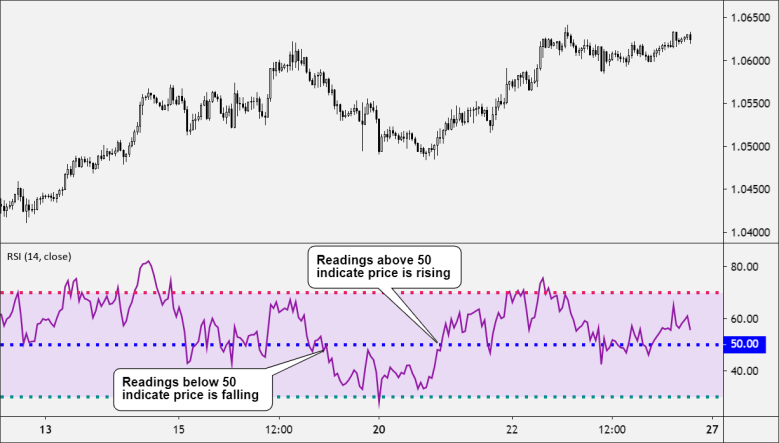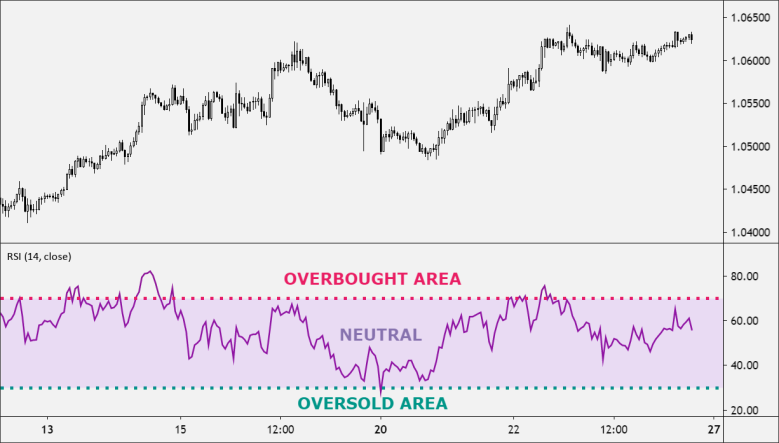Partner Center Find a Broker

The RSI or Relative Strength Index is a technical indicator that measures the strength or weakness of a currency pair by comparing its up movements versus its down movements over a given time period.

It does this by keeping track of recent price gains and losses and compares them to the current price.

The RSI indicator was created by J. Welles Wilder Jr. and first appeared in 1978, in his  book, “New Concepts in Technical Trading Systems.”

The RSI is considered a momentum indicator which means that it’s used to determine the speed and strength of price movement and whether the underlying momentum is strengthening or weakening.

Aside from helping traders identify price momentum, RSI is also used to identify overbought and oversold conditions, as well as divergence and hidden divergence signals

The RSI is displayed as an oscillator (a line graph that fluctuates between two extremes) and oscillates between 0 and 100.On a price chart, the RSI indicator is plotted as a single line that is calculated by combining the following information over a given period:

• The average gain during advances within a set time period.
• The average loss during declines within a set time period.

A ratio of these two values is used to create a measure that moves between 0 and 100.

• Readings over 50 indicate price movement that is generally rising,
• Readings below 50 indicate price movement that is generally falling.The RSI is considered “oversold” when below 30 and  “overbought” when above 70 so there are three primary “areas” to consider:

1. 0-30: Oversold (OS) area
2. 30-70: Neutral area
3. 70-100: Overbought (OB) areaThe default period setting for the Relative Strength Index (RSI) is 14 periods.

Traders do use different values, typically ranging from as low as 2 periods (for weekly charts) to as high as 25 periods (for shorter-term timeframes).

The Relative Strength Index (RSI) can be useful in a variety of different ways. It can be used:

• To confirm a new trend.
• To suggest when a recent price move might be reaching “overbought” and “oversold” levels.
• To warn of a potential price reversal due to a divergence between the actual price and the RSI indicator.

Let’s go over the different approaches to using RSI to generate trading signals.

Overbought/Oversold (Trend Reversal)

As price falls, RSI will head towards 0. In contract, as price rises, RSI will head towards 100. The more extreme the values, the more “overbought” or “oversold” the currency pair is considered to be.

• The upper range above 70 indicates overbought conditions.
• The lower range below 30 indicates oversold conditions.

An overbought signal suggests that the recent price rally may be ending (for the time being) and price might soon decline.

An oversold signal suggests that the recent price decline may be ending (for the time being) and price might soon rebound.

Convergence/Divergence (Trend Strength/Weakness)

• Convergence: When RSI follows in the SAME direction of the price, this signals potential trend strength and increasing bullish momentum.
• Divergence: When RSI moves in the OPPOSITE direction of the price, this signals potential trend weakness and decreasing bullish momentum.

When RSI reaches an overbought or oversold level, you should treat it as a warning alert that the current trend may soon run out of momentum.

Just because RSI moves into an overbought or oversold level does NOT guarantee a trend reversal will occur, only that the possibility exists.

Oversold signal (Trend Reversal)

A buy signal occurs when the RSI falls to an oversold level (30 or less) and then rises back above 30.

A buy signal occurs when the RSI is previously below 50 and then rises back above 50.

Bullish Divergence signal (Trend Reversal)

A buy signal occurs when a bullish divergence between the price chart and the RSI indicator forms. A bullish divergence is when RSI makes a higher low while price makes a lower low.

SELL Signals

Overbought signal (Trend Reversal)

A sell signal occurs when the RSI rises to an overbought level (70 or more) and then falls back below 70.

A sell signal occurs when the RSI is previously above 50 and then falls back below 50.

Bearish Divergence signal (Trend Reversal)

A sell signal occurs when a bearish divergence between the price chart and the RSI indicator forms. A bearish divergence is when RSI makes a lower high while the price makes a higher high.

How to Calculate RSI

Calculating RSI is a multi-step process and involves measuring relative strength by comparing the average periodic gains and losses.

This is done by the following:

• Average Gain: A gain is a positive change in periodic closing prices. To calculate the average gain, all periodic gains are added and then divided by the period itself (Total Gain / Period).
• Average Loss: A loss is a negative change in periodic closing prices. To calculate the average loss, all periodic losses are added and then divided by the period itself (Total Losses / Period).
• Relative Strength (RS): Relative Strength is derived by dividing the average gain by the average loss (Average Gain / Average Loss).

Lett’s take a look at the RSI formula using the default 14-period setting:

RSI = (100 – (100 / (1 + RS)))

In the formula above, RS stands for “Relative Strength”.

Now we need to calculate the value of the Relative Strength (RS).

RS = (14 EMA on the last 14 up bars) / (14 EMA on the last 14 down bars)

After you determine the value of the RS, you can apply the result in the first formula. This will give you the current RSI value.